Next: 3 Cryocrystals as Samples Up: 2 Muon Spin Rotation Previous: 2.2.1 Time-Differential

# 2.3 Data Reduction

We are interested in extracting the asymmetry, the signal manifesting the influence of the muon's environment on its spin. Ultimately we will want to fit the experimental asymmetry to a model function in order to measure some physical property, but ideally the experimental asymmetry should be obtained independently of the model function. One way of extracting the signal from the raw data is to make use of the symmetry of the detectors on opposite sides of the sample. With this geometry the two detectors view the same positron angular distribution, but from diametrically opposed directions, so that the counts in the two histograms are (for the Back-Front pair)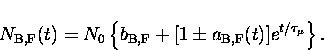(7)
We can then calculate the asymmetry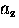for the (B,F) pair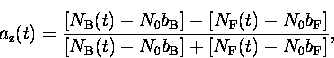(8)
naively assuming a common asymmetry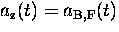.Ideally, the efficiency (probability of a particle being detected) of all positron detectors would be the same, as would be the solid angle they subtend. In general neither of these actually holds, so corrections are needed to avoid distorting the signal. It is easy to see, for example, that if the sample were closer to the B positron detector than to F, then B would intercept (all other things being equal) more positrons by virtue of its larger solid angle, and because it averages over a greater range in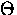, the amplitude of the precession signal in our TF experiment would be lower. Photomultiplier tube characteristics, operating voltages and discriminator settings will have a direct bearing on the efficiency of each detector. It would be a difficult task to match a pair of detectors so they had identical characteristics; fortunately, it is not necessary to do so. If we allow for differing efficienciesand asymmetries Ai Pz(t), where Pz(t) is the spin polarization along the z-axis, which is common to the Back and Front detectors, then each histogram is described by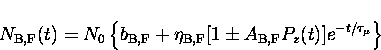(9)
and the experimental asymmetry is now

in which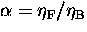and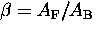.Rearranging this we obtain a function of the experimental asymmetry a(t) corrected for differences between detectors.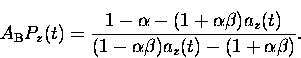(10)
This introduces two free parameters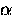and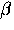that can be obtained by fitting a model spin polarization function to the experimental asymmetry. The same procedure can be carried out for the other pair of detectors on the x or y axes. (Usually there is no need to use detectors on more than two axes since there can never be any asymmetry along an axis that is always perpendicular to the muon spin.)

Returning to our example TF experiment, Fig. 2.7 shows the corrected asymmetry extracted from the (B,F) pair of detectors.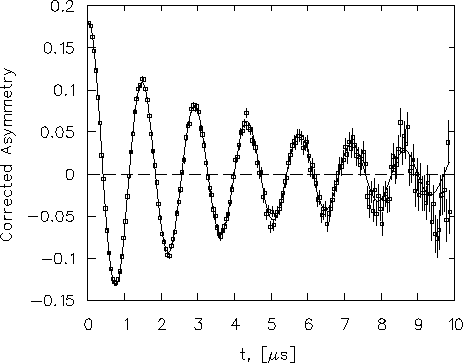The evolution of the muon spin polarization is essentially the same as the familiar free induction decay signal of nuclear magnetic resonance. The most obvious characteristic is the Larmor precession frequency, giving the mean magnetic field at the muon site. We will need to draw on everything we know about the sample from other sources in order to interpret the polarization relaxation function. For example, if we know the sample contains atoms possessing magnetic moments then we expect the muon will be subject to internal fields which may fluctuate in time or in space (or both). In this case, muons will experience different local fields and so precess at different frequencies, which would be reflected in the loss of coherence of the precessing muon ensemble. In other cases, the disappearance of polarization may be entirely due to one or more chemical reactions. The loss of polarization then simply reflects the amount of the muon ensemble that has undergone a chemical reaction. This must be the case for the signal seen in our Ne sample, where there are no nuclear magnetic moments present. The amplitude of the muon signal decreases with time because the muons pick up stray electrons and so form neutral muonium atoms (a topic that will occupy much of our attention in chapters to follow) which behave entirely differently - the diamagnetic" muon signal gradually vanishes with time. In either case we need to have some idea qualitatively what may be happening to the muon before we can extract quantitative information by fitting a model polarization function. Measuring the polarization function is the easy part; deducing the correct model is usually challenging, but yields all the physics.

We have discussed here only the most common sorts of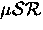experiments. Other techniques, falling under the general label of Time-Integral, are described elsewhere, [5,6] including level-crossing resonance experiments and RF-.Next: 3 Cryocrystals as Samples Up: 2 Muon Spin Rotation Previous: 2.2.1 Time-Differential